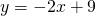# Solution assignment 06 Tangent line to graph

### Assignment 6

Calculate the equation of the tangent line at the point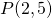of the graph of the function: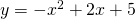### Solution

First we calculate the slope of the tangent line at the given point and we do this by differentiating the function: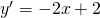In the pointthe slope is (always check whether such a point lies on the graph):So we have to calculate the equation of the line throughwith slope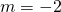. We find the line by using the following formula: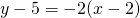or: